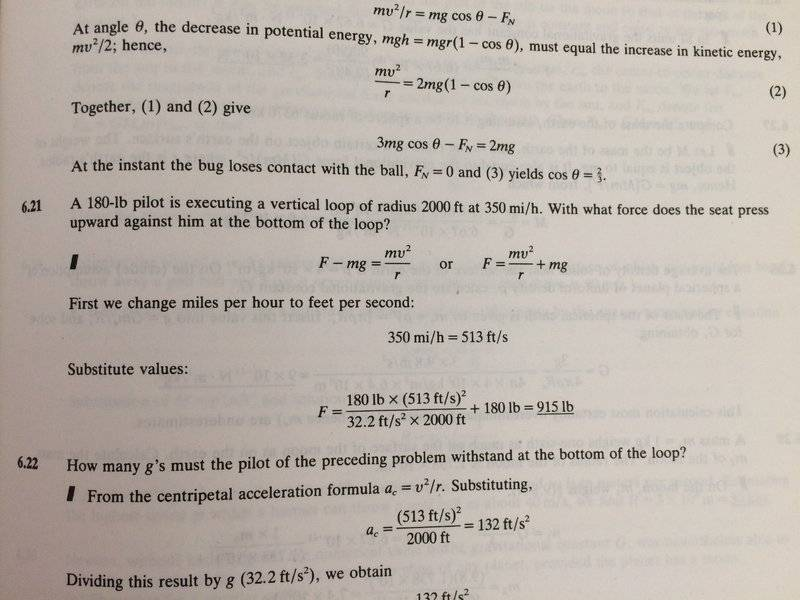# Circular motion: normal force on a loop

• Beth N
In summary, the conversation discusses a discrepancy in an answer key for a problem involving a pilot flying a vertical loop. The discrepancy is due to the use of lb-force and lb-mass units, and it is resolved by using the correct value for mass in the equation. The conversation also mentions the use of slugs as a unit for mass in Imperial units.f

## Homework Statement

A 180lb pilot flies a verticle loop with radius 2000 ft at 350 mi/h. With what force does the seat fress upward against him at the bottom of the loop?
Problem 6.21## Homework Equations

##F=ma##
##F_c=\frac {mv^2} {r} ##

## The Attempt at a Solution

There seems to be a discrepancy in the answer key provided? On the first line the equation they came up is ## F= \frac {mv^2} {r} + mg## . But when the number was plugged in, it seems like they use the equation ##F= \frac {mv^2} {gr} + m ## Which equation is correct? My own answer corresponds with the first equation, which is why I get a different numerical answer.

Thank you!

#### Attachments

Are you familiar with the difference between lb-force and lb-mass? What is the weight (in lb-force) of a body that has a mass of 180 lb-mass?

•Beth N
I'm more familiar with the unit with kilogram. But I guess the weight in lb-force would be mass in lb * 32.2 ft/second^2 ? (as opposed to 9.8 m/second^2 for kg). So the weight in lb-force of a body with mass 180 lb is 5796 lb? Still, I haven't quite understood the discrepancy in the answer key.

Oh wait I get what you are saying now. 180-lb as indicated in the book's question is the weight (m*a), not the mass (m). I didn't realize that. Thank you!

I'm more familiar with the unit with kilogram. But I guess the weight in lb-force would be mass in lb * 32.2 ft/second^2 ? (as opposed to 9.8 m/second^2 for kg). So the weight in lb-force of a body with mass 180 lb is 5796 lb? Still, I haven't quite understood the discrepancy in the answer key.
You really should use lb only for the mass unit and lbf for the force unit. The weight of a body with mass x lb in standard gravity is by definition x lbf, not 32.2x lbf. This is by definition of the pound force unit. There is also a similar kg related unit that sees very little use, kgf.

•Beth N
In Imperial units, the weight of 1 lb-mass is 1 lb-force. Crazy, huh? This all resolves itself when we specify that the mass to use in applying Newton's second law using Imperial units is the slug, which is the mass in lb-mass divided by 32.2.

•Beth N
Yes, but be sure you use the correct value for mass in the mv^2/r term!